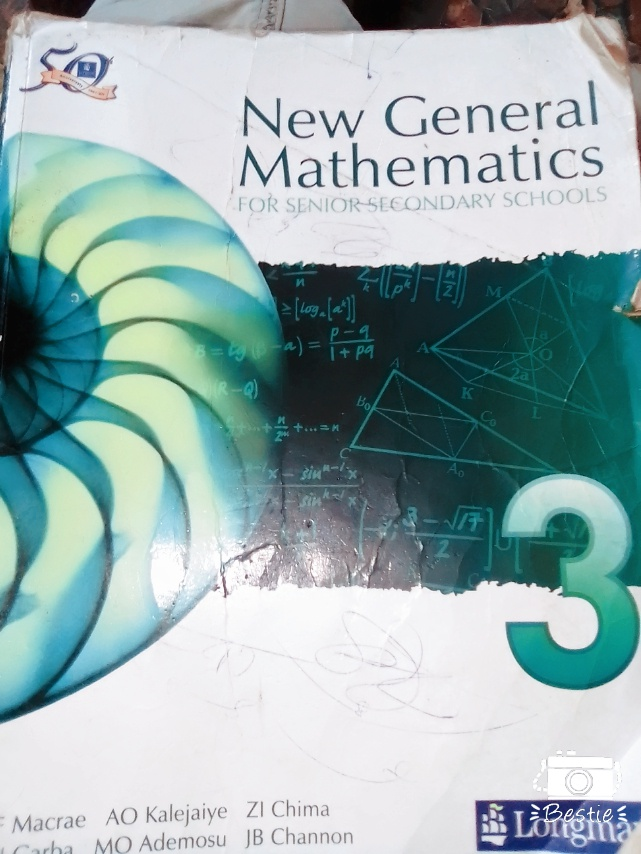#The Subject of mathematics no doubt has over time come to be seen by a lot of students as a very difficult subject. Sadly, many of such students fail to realise that such fears can be addressed successfully. A student who works hard at the following topics in mathematics will come out with flying colours. The major topics at SSCE are:

1, Bearing.

2,Geometric Construction and loci.

3, Problems on latitudes and longitude.

4, Mensuration.

5, Logarithms.

6, Indices.

7, Statistics and Probability.

8, Trigonometry.

9, Surd.

10, Equations-(simple linear equations, simultaneous equations and quadratic equations)

11, Variation.

12, Inequalities.

13, Simple Interest.

14, Compound Interest.

15, Graphs.

16, Sequences-( A.P) Arithmetic Progression in which the nth term is given by a+(n-1)d where a = the first term , n= the number of terms and d = common difference.(G.P) Geometric Progression in which the nth term is given by the product of “r” raised to the power of (n-1) and a .